# Area Model Multiplication – Definition With Examples

Home » Math-Vocabluary » Area Model Multiplication – Definition With Examples

## What Is Area?

To understand the area model in math, we must understand the meaning of area first. The space occupied by a flat shape or surface of an object is known as area. The area of the rectangle given below is the shaded part. Area of a rectangle is the base of the area model of solving multiplication and division problems.

Area of a rectangle $= \text{Length} \times \text{Width}$

If the rectangle has a length equal to 25 units and width 18 units, then this area can be calculated by finding the product $25 \times 18$. In other words, when you consider the product $25 \times 18$, geometrically, we can interpret it as the area of a rectangle of length 25 units and width 18 units.

## Area Model of Fractions

A fraction area model represents a whole shape that is split into equal parts.

Suppose we are given a model representation of a fraction. To identify the fraction, we use the following steps:

• Step 1: The whole shape is divided into equal parts. We count these numbers. We write this number as the denominator. In this example, there are 3 equal parts, so the denominator is 3.
• Step 2: Count the number of shaded shapes given in the figure. We write this number as your numerator. There are 2 shaded parts. So, the numerator is 2.
• Step 3: We write it in the form of $\frac{Numerator}{Denominator}$. In this example, we get $\frac{2}{3}$.

## What Is an Area Model of Multiplication and Division?

In mathematics, an area model is a rectangular diagram that is used to multiply and divide two numbers or expressions, in which the factors or the quotient and divisor define the length and width of the rectangle. We can break down one large area of the rectangle into several smaller boxes, using number bonds, to make the calculation easier. Then we add to get the area of the whole, which is the product or quotient.

As mentioned earlier, Area of a shape is the space occupied by the shape. The area of the given rectangle is the shaded part.

If the rectangle has a length equal to 25 units and width 18 units, then this area can be calculated by finding the product $25 \times 18$. In other words, when you consider the product $25 \times 18$, geometrically we can interpret it as the area of a rectangle of length 25 units and width 18 units.

Further, we can divide this rectangle into smaller rectangles for easier calculations, and their areas can be added to get the total area of the rectangle. We can do this by breaking the lengths of the sides into smaller numbers using expanded forms. For example, 25 can be written as $20 + 5$. Similarly, the width 18 can be written as $10 + 8$. Using these, we can divide the original rectangle into 4 smaller rectangles as shown.

Now, it is easier to calculate the areas of these smaller rectangles and their sum gives the total area or the product $25 \times 18$.

The rectangle I has a length of 20 units and width of 10 units making an area of 200 square units. Similarly, the rectangle II has an area equal to 10 times 5 or 50 square units. Likewise, we can calculate the areas of rectangles III and IV as 40 sq. units and 160 sq. units respectively.

Now, the total area of the rectangle of sides 25 units and 18 units is calculated by adding these partial sums.

$200 + 50 + 40 + 160 = 450$ sq. units

One can check using the standard algorithm to multiply two 2-digit numbers that 25 times 18 is 450.

In a rectangular auditorium, there are 36 rows of chairs with 29 chairs in each row.

To find the total number of chairs we need to find the product 36 × 29.

Step 1: Write the multiplicand and the multiplier using expanded forms.

$36 = 30 + 6$

$29 = 20 + 9$

Step 2: Find the areas of the smaller rectangles.

Step 3: Add the partial sums to get the total area.

Thus, there are 1044 chairs in the auditorium.

## Area Model of Multiplication of Whole Numbers

Let’s understand the steps for the area model of multiplication with respect to different cases.

## Multiplication of Two-digit Number by One-digit Number

Example: $65 \times 7$

• Step 1: Write the multiplicands in expanded form.

In this example, $65 = 60 + 5$

• Step 2: Draw a $2 \times 1$ grid or 1 row and 2 column box.
• Step 3: On the top of the grid, write “the tens and ones part of the multiplicand we expanded earlier. On the left side we write the other expanded multiplicand. In this example:
• Step 4: In the first cell, write the product of the number 7 and the tens part of the number 65. In the second cell, write the product of the number 7 and ones part of 65. In this example:
• Step 5: Add the partial products i.e. numbers in each of the cells. In this example:

$420 + 35 = 455$

So, we can say that $65 \times 7 = 455$

## Multiplication of Two-Digit Number by Two-Digit Number

Example: $52 \times 79$

• Step 1: Write the multiplicand and multiplier, i.e., 52 and 79 in expanded form.

In this example, $52 = 50 + 2 and 79 = 70 + 9$

• Step 2: Draw a grid of size 2 by 2.
• Step 3: On the top of the grid, write the expanded terms for one of the multiplicands as shown in the previous example. Mention the same for the other multiplicand on the left side.
• Step 4: In the first cell, put down the product of the tens of both the numbers. In the second and third cells, put the product of the tens and ones of the numbers accordingly. In the fourth cell, put the product of the ones of both the numbers.
• Step 5: Add the partial products. In this example:

$3500 + 140 + 450 + 18 = 4108$

So, we can say that $52 \times 79 = 4108$

## Multiplication of Three-Digit Number by Two-Digit Number

In the multiplication of a 3-digit number by a 2-digit number, we make a grid of $2 \times 3$.

For example: Multiply $248 \times 81$

$248 = 200 + 40 + 8$ and $81 = 80 + 1$

Adding the partial products, we get $16000 + 3200 + 640 + 200 + 40 + 8 = 20088$

So, the product $248 \times 81 = 20088$

## Area Model of Multiplying the Fractions

Proper Fractions

We can also multiply two proper fractions using an area model. Suppose we have to multiply $\frac{3}{4}$ and $\frac{1}{2}$, we will follow the steps given below:

Step 1: Draw a rectangle and mark length on one side and breadth on the other side. In this case, mark $\frac{3}{4}$ as length and $\frac{1}{2}$ as breadth as $\frac{3}{4} \gt \frac{1}{2}$.

Step 2: Look at the length. Divide the length into the parts as much as the denominator and shade the parts same as the numerator. In this case, divide the length into 4 equal parts and shade 3 out of them.

Step 3: Look at the breadth. Divide the breadth into the parts as much as the denominator and shade the parts the same as the numerator. In this case, divide the breadth into 2 equal parts and shade 1 out of them.

Step 4: See the common shaded parts. In this case, there are three shaded parts, so the numerator of the product is 3.

Step 5: See the total number of equal parts. In this case, the total number of equal parts are 8. So, the denominator of the product is 8.

Step 6: Write the product fraction as $\frac{Numerator}{Denominator}$. In this case, the product $= \frac{3}{8}$.

## Area Model for Multiplying the Decimals

Suppose we have to multiply 3.6 and 2.2.

Step 1: Break the decimal into a whole number and decimal portion.

Here, $3.6 = 3 + 0.6$ and $2.2 = 2 = 0.2$

Step 2: Make a $2 \times 2$ grid

Step 3: On the top of the grid, write one of the multiplicands, and on the left side of the grid, write the other one, as mentioned in the image.

Step 4: Multiply the whole part of one multiplicand with both the parts of the second multiplicand and fill the first row. Here, we are first multiplying 2 and 3 and then, 2 and 0.6.

Step 5: Multiply the decimal part of the same multiplicand with both the parts of the second multiplicand and fill the second row. Here, we are multiplying 0.2 and 3 and then, 0.2 and 0.6.

Step 6: Add the numbers in all 4 cells to find the answer.

So, $3.6 \times 2.2 = 6 + 1.2 + 0.6 + 0.12 = 7.92$

## Area Model of Division of Whole Numbers

Area of a rectangle with the length of 48 units and a width of 21 units can be measured by multiplying 48 by 21. Similarly, a division problem like $352 \div 22$ can be represented geometrically as the missing dimension of a rectangle with an area of 352 square units and a side of 22 units. The division is considered to be complete when the difference we get at the end is less than the divisor. The difference we are left with at the end is known as the remainder.

## Division without Remainder

Suppose we have to divide 432 by 16.

• Step 1: Write the dividend inside the box and the divisor outside the box.

In this example:

• Step 2: We will find a number divisible by 16 and less than or equal to 43. We get 32. We will add 0 after it. After eliminating 320 from 432, we get 112. And we will write quotient on the top, i.e., 20.
• Step 3: We move to the next box. Now we find a number divisible by 16 and less than equal to 112. We get $16 \times 7 = 112$.

We get the answer: $432 \div 16 = 20 + 7 = 27$

Another example of area model of division is given below:

## Fun Facts

• The area model is also known as the box model.
• This model of multiplication uses the distributive law of multiplication.
• Expanded forms are used to multiply numbers with more than 1 digit.
• The area model of solving multiplication and division problems is derived from the concept of finding the area of a rectangle.
Area of a rectangle $= \text{Length} \times \text{Width}$

## Solved Examples

1. What fraction is given in the figure?

Solution: The denominator is 8. The numerator is 4.

So, the fraction is $\frac{4}{8}=\frac{1}{2}$.

2. Calculate the product of 48 and 6 by area model.

Solution:

Alt tag: Product using area model

$48 \times 6 = 240 + 48 = 288$

3. Find the product of 39 and 42 using the area model.

Solution:

$39 \times 42 = 1200 + 360 + 60 + 18 = 1638$

4. Divide 5607 by 5 using the area model and write the quotient and remainder.

Solution:

Quotient $= 1000 + 100 + 20 = 1120$ and remainder $= 2$

5. Multiply $\frac{3}{5}$ and $\frac{2}{3}$ using an area model.

Solution:

Total mutual shaded parts $= 6$

Total equal parts $= 15$

Fraction $= \frac{6}{15} = \frac{2}{5}$

## Practice Problems

1

### Which of the following model is not the correct representation of the fraction $\frac{3}{5}$?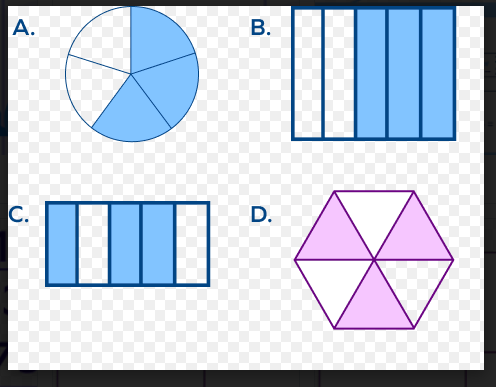A
B
C
D
CorrectIncorrect
D
2

### What will be the value of the blank in the given model for division to find $4870 \div 79$ ?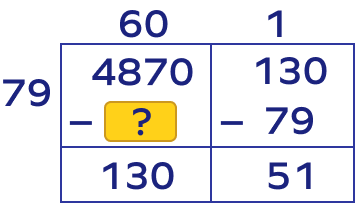4640
7960
4740
474
CorrectIncorrect
$79 \times 60 = 4740$
3

### What will be the missing number when we multiply 2.3 and 4.7?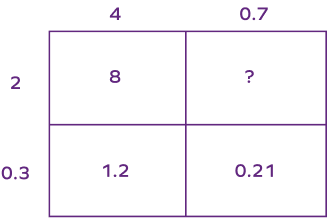14
1.4
0.14
0.014
CorrectIncorrect
We know $2 \times 0.7 = 1.4$
4

### What will be the missing multiplicands in the following model of multiplication?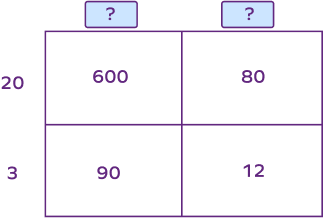60, 8
9, 2
30, 4
30, 40
CorrectIncorrect
30, 4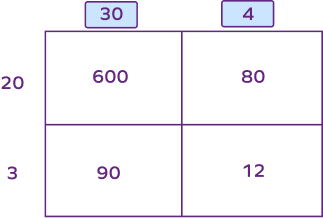5

### What would be the resultant fraction when two fractions are multiplied using the given model?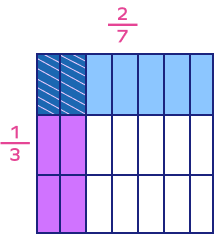$\frac{2}{7}$
$\frac{1}{3}$
$\frac{2}{3}$
$\frac{2}{21}$
CorrectIncorrect
Correct answer is: $\frac{2}{21}$
D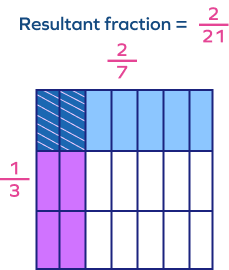Yes, we can use the area model to multiply polynomial expressions. Let’s look at the example given below. Here we are multiplying $(x + 2)$ and $(x + 4)$.